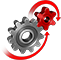# WolframSystemModeler

## Comparison: A Transient and Quasi-stationary DCPM Motor

An often important step in the development of system models is deciding on the appropriate level of model fidelity. In many cases, such a decision becomes a tradeoff between model accuracy—that is, how well the model will be able to describe the behavior of the real‐world system—and simulation speed and performance. Here, we study how a fast (quasi-stationary) model of a direct current permanent magnet (DCPM) motor behaves differently compared to a fully transient one.

### A Comparative Model

The model is built using components from the Modelica Standard Library. The motor models can be found in the Modelica.Electrical.Machines sub‐library.The model diagram. Both motors start at no-load speeds, and then torque pulses are applied to their respective shafts.

### Simulation Results

Quasi-stationary analyses of electrical circuits apply complex phasors, or sinusoidal excitations, with known frequency, amplitude, and phase shift. All dynamic transients, which are considered in a transient analysis, are neglected. This behavior can be seen in the simulation results from the model.The upper plot shows the armature current of the two motors, and the bottom plots the electrical torque and angular velocity of the rotor against stator. As is clear from these plots, all transient effects are absent in the quasi-stationary model.

Compare different types of electrical models

Create a comparative model to analyze the difference between a quasi-stationary and a transient DCPM motor.

## Wolfram System Modeler

Questions? Comments? Contact a Wolfram expert »